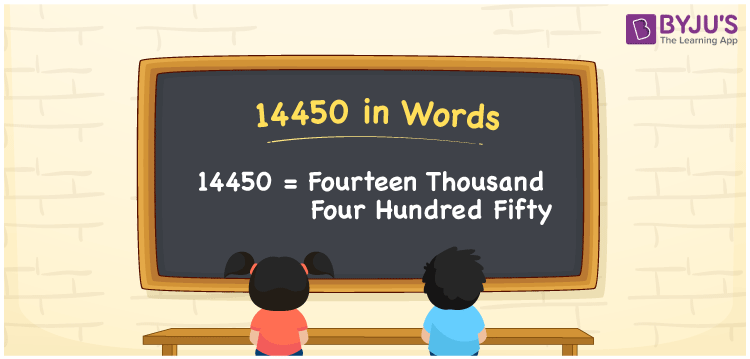# 14450 in words

14450 in words is written as Fourteen Thousand Four Hundred and Fifty. In 14450, 1 has a place value of ten thousand, first 4 is in the place value of thousand, second 4 has a place value of hundred and 5 is in the place value of ten. The article on Place Value gives more information. The number 14450 is used in expressions that relate to money, distance, social media views, area and many more. For example, “A new car model had Fourteen Thousand Four Hundred and Fifty buyers in the month of January”.

 14450 in words Fourteen Thousand Four Hundred and Fifty Fourteen Thousand Four Hundred and Fifty in Numbers 14450

## 14450 in English Words## How to Write 14450 in Words?

We can convert 14450 to words using a place value chart. The number 14450 has 5 digits, so let’s make a chart that shows the place value up to 5 digits.

 Ten thousands Thousands Hundreds Tens Ones 1 4 4 5 0

Thus, we can write the expanded form as:

1 × Ten thousand + 4 × Thousand + 4 × Hundred + 5 × Ten + 0 × One

= 1 × 10000 + 4 × 1000 + 4 × 100 + 5 × 10 + 0 × 1

= 14450.

= Fourteen Thousand Four Hundred and Fifty.

14450 is the natural number that is succeeded by 14449 and preceded by 14451.

14450 in words – Fourteen Thousand Four Hundred and Fifty.

Is 14450 an odd number? – No.

Is 14450 an even number? – Yes.

Is 14450 a perfect square number? – No.

Is 14450 a perfect cube number? – No.

Is 14450 a prime number? – No.

Is 14450 a composite number? – Yes.

## Solved Example

1. Write the number 14450 in expanded form

Solution: 1 x 10000 + 4 x 1000 + 4 x 100 + 5 x 10 + 0 x 1

Or Just 1 x 10000 + 4 x 1000 + 4 x 100 + 5 x 10

We can write 14450 = 10000 + 4000 + 400 + 50 + 0

= 1 x 10000 + 4 x 1000 + 4 x 100 + 5 x 10 + 0 x 1.

## Frequently Asked Questions on 14450 in words

Q1

### How to write the number 14450 in words?

14450 in words is written as Fourteen Thousand Four Hundred and Fifty.
Q2

### State whether True or False. 14450 is divisible by 3?

False. 14450 is not divisible by 3.
Q3

### Is 14450 divisible by 10?

Yes. 14450 is divisible by 10.